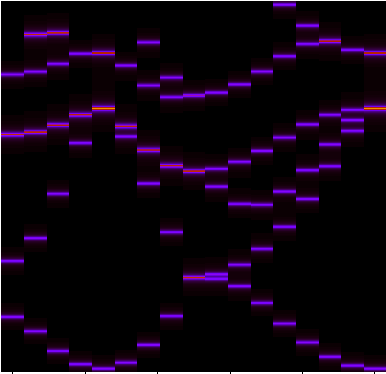# Unfolding

In this tutorial, we look at how to perform a band-structure unfolding using Octopus.

This calculation is done in few steps, which are described below. First, we need to compute the ground state of a supercell.

## Input

Here we start with the input file for Octopus. This is a ground state calculation for bulk silicon, similar to the one of the tutorial Periodic Systems. In the present case however, we will use a supercell of Si, composed of 8 atoms.


CalculationMode = gs

PeriodicDimensions = 3
BoxShape = parallelepiped
Spacing = 0.5

a = 10.18
%LatticeParameters
a | a  | a
90 | 90 |90
%

%ReducedCoordinates
"Si" | 0.0         | 0.0       | 0.0
"Si" |   1/2       | 1/2       | 0.0
"Si" |   1/2       | 0.0       | 1/2
"Si" |   0.0       | 1/2       | 1/2
"Si" |   1/4       | 1/4       | 1/4
"Si" |   1/4 + 1/2 | 1/4 + 1/2 | 1/4
"Si" |   1/4 + 1/2 | 1/4       | 1/4 + 1/2
"Si" |   1/4       | 1/4 + 1/2 | 1/4 + 1/2
%

nk = 4
%KPointsGrid
nk |  nk |  nk
%
KPointsUseSymmetries = yes


Most of these variables are already used in other tutorials, so the interested read could refer for instance to Getting started with Periodic Systems for a detailed description.

## Unfolding - step 1: Setup

After running Octopus using the previous input file, we have the obtained the ground state of the supercell. We now want to define the primitive cell on which we want to unfold or supercell, and the specific k-point path that we are interested in.

This is done by adding to the input file the following lines:


UnfoldMode = unfold_setup
%UnfoldLatticeParameters
a | a | a
%
%UnfoldLatticeVectors
0.  | 0.5 | 0.5
0.5 | 0.  | 0.5
0.5 | 0.5 | 0.0
%
%UnfoldKPointsPath
4 | 4 | 8
0.5 | 0.0 | 0.0 # L point
0.0 | 0.0 | 0.0 # Gamma point
0.0 | 0.5 | 0.5 # X point
1.0 | 1.0 | 1.0 # Another Gamma point
%


Lets see more in detail some of the input variables:

• UnfoldMode = unfold_setup: this variable instruct the utility oct-unfold in which mode we are running. As a first step, we are runing in the unfold_setup mode, which generates necessary files for getting the unfolded band structure.

• UnfoldLatticeParameters specifies the lattice parameters of the primitive cell. This variable is similar to LatticeParameters.

• UnfoldLatticeVectors specifies the lattice vectors of the primitive cell. This variable is similar to LatticeVectors.

• UnfoldKPointsPath specifies the k-point path for the unfolded. The coordinates are indicated as reduced coordinates for the primitive lattice. This variable is similar to KPointsPath.

The unfolding still been an experimental feature, you also need to add to your input file the line


ExperimentalFeatures = yes


Expand for complete input file

By running oct-unfold, you will obtain two files. The first one (unfold_kpt.dat) contains the list of k-points of the specified k-point path, but expressed in reduced coordinates of the supercell. These are the k-points for which we need to evaluate the wavefunctions of the supercell. The second file (unfold_gvec.dat) contains the list of reciprocal lattice vectors that relate the k-points of the primitive cell to the one in the supercell.

Expand for the unfold_kpt.dat file
Expand for unfold_gvec.dat file

## Unfolding - step 2: Obtaining the wavefunctions

In order to perform the unfolding, we must compute wavefunctions in the supercell at specific k-points. These points are described in the file unfold_kpt.dat. To get them, we need to run an non self-consistent calculation using


CalculationMode = unocc


In order to use the list of k-points obtained in step 1, we remove from the input file the k-point related variables (%KPointsGrid, %KPointsPath, or %KPoints) and we replace them by the line


include unfold_kpt.dat


We then run Octopus on this input file. Note that in order to get extra states, one should use the variables ExtraStates and ExtraStatesToConverge, as for a normal band structure calculation, see Periodic Systems.

For this tutorial, we used


ExtraStates = 6
ExtraStatesToConverge = 4

Here, we are requesting to converge 4 unoccupied states, which all correspond to the first valence band in the folded primitive cell, as the supercell is 4 times larger than the initial cell.

Expand for complete input file

## Unfolding - step 3: Unfolding

Now that we have computed the states for the required k-points, we can finally compute the spectral function for each of the k-points of the specified k-point path. This is done by changing unfolding mode to be


UnfoldMode = unfold_run


This will produce the spectral function for the full path (static/ake.dat) and for each individual points of the path (static/ake_XXX.dat). The unfolded bandstructure of silicon is shown below.Unfolded band structure of bulk silicon.

Note that in order to control the energy range and the energy resolution for the unfolded band structure, one can specify the variables UnfoldMinEnergy, UnfoldMaxEnergy, and UnfoldEnergyStep.

It is important to note here that the code needs to read all the unoccupied wavefunctions, and therefore will need to have enough memory.